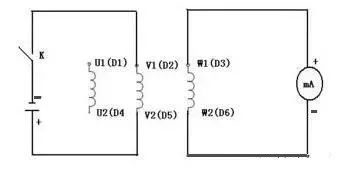# How to judge the front end of the motor?

24/08/2019You can use a multimeter to first distinguish six wire ends, divide the two wire ends that are connected into one phase, and the six wire ends can be divided into three phases, and then judge the head and tail of the winding.

The specific method: the DC amp of the multimeter is hit to the minimum one, and the test leads are connected to one end of one of the three-phase windings, and the positive and negative poles of the battery are connected to the two ends of the other phase.

As shown in the figure, when the switch is closed, if the direction of the hand is greater than zero, it means that the wire head connected to the negative pole of the battery and the end of the pen connected by the multimeter are the same name (both can be regarded as the head). By analogy, the head and tail of the other two phases can be measured.

Wiring diagram:Use a pointer multimeter to determine:
1. Select the multimeter gear position in the milliamp position;

2. Connect the three terminals of the stator coil of the motor in parallel;

3. Connect the two tables of the multimeter in parallel with the two ends of the good coil;

4. Turn the motor rotor by hand;

5, watch the multimeter pointer action:

a, if it is not moving, the first end connection of the motor is correct (because the rotor remanence in the three-phase coil induces the potential vector sum is zero);

b. If the motion is the wrong connection between the first and last ends of the motor (because the rotor residual magnetism induces a potential vector and is not zero in the three-phase coil), the coil terminal can be replaced gradually.